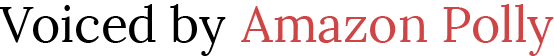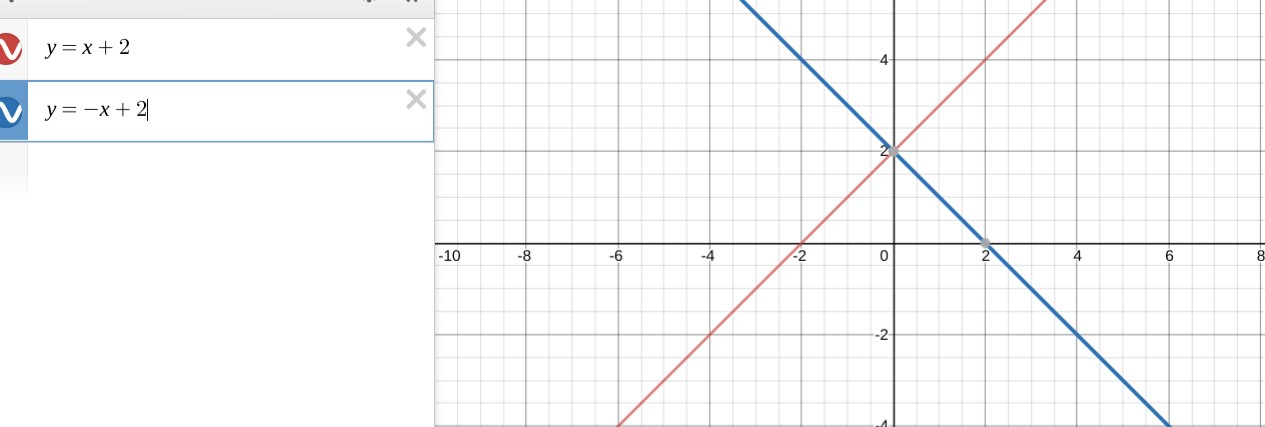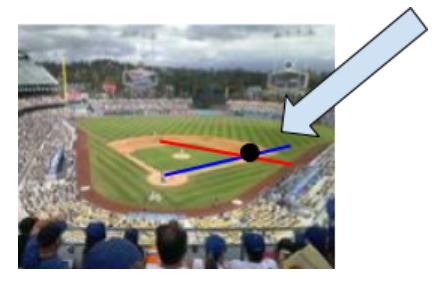# Solve Systems by Graphing

 Would You Rather Listen to the Lesson?When I coached peewee soccer, I remember putting dots or cones on the ground to help my players with their passing.  At first, it was easy.  One player stood at the cone and passed the ball to the second player who was standing at another cone.  We practiced short, crisp passes.  The shortest distance between two points is a straight line.  That ball scooted straight across.  After warming up, I talked about how in the game, everyone is moving.  We have to pick a point ahead of our teammate and pass the ball there.  That way, the player can run onto the ball, receive the pass and keep going.  To help practice this, the player with the ball tried to pass the ball to a cone.  However, the player receiving the ball started at a different spot and ran to that cone to catch the pass.  The receiver was running in a straight line, the pass was going in a different line, and if they met at the same point (the cone), the pass was successful!

When two lines cross at the same point, that’s solving a system of equations.  Two linear equations that cross create a solution to the system.  In order to solve a system by graphs, students must plot the equations and find the coordinate pair that they share.  That coordinate pair is the solution to the system – just like a successful pass!

If someone is running in one direction and I pass the ball parallel to them, will they be able to catch the pass?  No!  Because the ball and the player never cross paths.  In math, that’s called “No Solution”.  Or if I have the ball already, and I’m running down the field dribbling it, we are traveling along the same line.  I can constantly have touches on the ball, over and over and as long as the ball and I are on the same line, there is an infinite number of solutions.  This is the same as on a coordinate plane.  Two functions that lie on the same line share an infinite number of solutions.  Parallel lines, which will never cross, will never share a solution; and two lines that intersect have exactly one solution or point that they share.

If I have the time and the space, I get my students outside to play some catch.  I have used a soccer ball or basketball, a tennis ball or baseball.  The key is to show how the ball travels in a straight line and a person travels in a straight line.  If that person makes the catch, then they capture the solution of the system.  The point in space that those two lines share is how to solve a system.  Of course, if I don’t have a lot of space, I may try this with a soft ball or bean bag to show the paths and the lines that create the system.  Sometimes I show a video of a sports play, football or basketball, soccer or baseball.  You could even have students fold paper into triangles and flick it with their pointer finger to “pass it.” We can draw lines to show the path of the ball.  The key is to show that in order to solve a system by graphing, we have to find the coordinate pair that the lines share.

## Set up for Solving Systems by Graphing Activity

1. To introduce the activity with a demonstration, prepare by finding a ball that works for the space you will do this in. Consider the type of ball that works for outside (soccer ball, basketball, baseball) vs inside (tennis ball, beach ball, squishy ball).
2. Prepare a video of a football play, basketball or soccer passing drill, or a baseball play where the runner gets out at the first base.  You can draw a model of this on the board for the class.  (Finding drill videos or replays that are marked up can be helpful too.) Here are some ideas you can use and discuss: Soccer Passing  and Close Calls, Plays to first Base Video
3. Each student needs a  Solving Systems by Graphing Worksheet. with solving systems by graphing examples and solving systems of equations activity.
4. Organize partnerships so students can work in pairs.

## Launch the Graphing Activity

Pair off your students and ask for a group to demonstrate the idea behind “Complete the Pass”.  Set it up like a drill in baseball or soccer.  One player has the ball and is throwing it to a spot.  The other player is moving towards that same spot and trying to catch the pass.

On a coordinate plane, have students sketch the two lines that the ball and the runner made. Let the groups try on their own and sketch their system of equations.

Explain that a system of equations is two or more equations on the same coordinate plane. Have everybody sketch the linear equation: y=x+2. You can review how to plot the graph by knowing y=mx+b, or by plugging in values.  The y-intercept is 2, because when x=0 y=2.  Plot this point together.  Then students can march the slope of 1, or plug in another value.  For example, when x= 1, y=3.  Plot points and draw the graph.  Explain that when they have a linear equation, any point on the line is a solution to that equation.

Now, on the same coordinate plane, plot the graph y= -x + 2. Again, students can work together and plot the graph by finding points or by using y=mx+b. The graph will look like this (Image Graphed using Desmos.com):Once students have a graph of the system:

y=x+2

y=-x+2

You can explain that every point along the red line is a solution to y=x+2.

Then go to the blue line and explain that every point along the blue line is a solution to the equation y=-x+2.

Ask “So is there a point that is a solution to this system?”

If the students are not sure, have them talk to each other about it.  Remind them about how a complete pass was made when the ball and the player were moving along different lines.  You can prompt with questions like “Do these two lines share a point?  Or share a solution?”

Place a dot where the lines cross.  The point (0,2).  On a graph, this is where they cross.  They share this point in space.  You can then explain how if they plug in the point (0,2) to the first equation, it is true and is a solution.  Then try to plug it into the second equation to show that it works for that line as well. The point (0,2) is a solution to BOTH equations.  Which means it is also a solution to the system of equations.

Help students with solving systems of equations by graphing step by step.

Before setting the students off to work together, show a quick video clip of some baseball plays, such as Video of Close Calls at First,  and diagram the system on the boards, like this:

The runner must run from home to first base.  The fielder must pick up the ball and throw it directly to first base.  Show these two lines on a coordinate plane.  The coordinate pair for the first base is where the throw and runner intersect.  This shows solving systems by graphing.

Example:Note – if you could find a field like this, draw a line for the runner, going from home to first base, and then a different color line for the throw going from shortstop to first base, and show how it is a linear system of equations.  The point where they cross is at first base.

Similar to the image belowAfter the demos and introduction, students will work on matching equations to “Complete the Pass” on the Solving Systems by Graphing Worksheet.

Explain that they will graph the first equation and then be given a point on the line.  That is where they must make the pass.  Can they find the equation that completes the pass?  The equation that will cross at that point?

Students will then solve the system by graphing.  They will graph the system of the 2 chosen equations and name the solution to the system.

Students should complete the demonstration graphs, introductory graph, and partner graphs on the Solving Systems by Graphing Worksheet.

## Complete the Pass: Solving Systems by Graphing Activity

1. Students work with a partner.
2. Students follow the Solving Systems by Graphing Worksheet.

ROUND 1 – Hit the Target, Make the Pass

1. Students graph the “Runner” Equation in BLUE.  Make sure the students choose at least 2 points or a graph with the y-intercept and slope.
2. Students then mark the indicated “catching” coordinate with an X.
3. Students choose from the 3 “Passer” Equations to find the line that solves the system.

ROUND 2: Choose the BEST line to complete the pass.

1. Students draw the “runner” graph.
2. There is no target, students must choose the equation that will complete the pass.
3. Students find the solution to the system of equations by graphing.
4. Students check the coordinates to see that it is a solution to the equation.

## Reflecting on the Solving Systems by Graphing Activity

Students should compare graphs and discuss the possible options with each other.

Ask students if they can find any patterns.  Prompt the discussion with the following questions:

• Can you tell if the line will not cross?
• What does it mean if you graph 2 lines that are parallel?
• What does it mean if you graph 2 lines, and they are actually the same line?
• What patterns did you discover with the lines that crossed?
• Can two lines with a positive slope cross? Explain.
• Can two lines with negative slopes cross?

## Extensions

1. Play a round of Complete the Pass by providing one equation and a “target” point.  Students must use the point to create 3 different equations that will also go through that point and solve the system.  Show the graph, and color code the linear equations.
2. Draw or print out a picture of a sports field or court.  Highlight the lines to discuss the system of equations.  Place your system on a coordinate grid or overlay a grid over your picture.  Write equations for each line and solve the system by graphing.